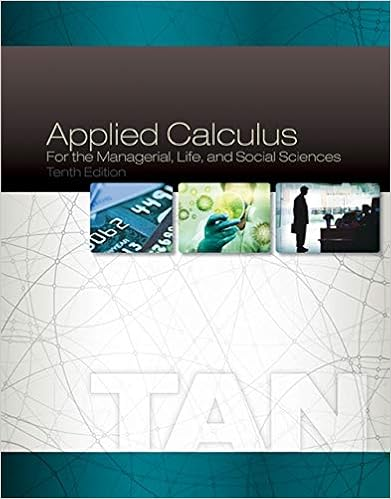Lecture Notes SMTH000 2019-1.pdf - CHAPTER 00 ALGEBRA...

• Notes
• 191

This preview shows page 1 - 3 out of 191 pages.

We have textbook solutions for you!
The document you are viewing contains questions related to this textbook.The document you are viewing contains questions related to this textbook.
Chapter 4 / Exercise 29
Applied Calculus for the Managerial, Life, and Social Sciences
TanExpert Verified
1 CHAPTER 00 ALGEBRA REFRESHER 0.1 PURPOSE This chapter, designed for your convenience, is a review of many pertinent topics from algebra. For the most part, your prior exposure to this material is assumed. Our intention is neither to develop nor to motivate any concepts; rather, we shall provide a statement-of-fact presentation of terminology and the methods of manipulative mathematics to which an immediate second exposure would be beneficial. Therefore, we urge you to devote whatever time necessary to these sections in which you need review. 0.2 AXIOMS OF THE REAL NUMBERS Certain fundamental principles, which we assume to be true, govern the system of real numbers. They are known as axioms (or laws) of the real number system. Axioms of Equality Let a, b and c be real numbers. Then 1. a = a (reflexive) 2. If a = b, then b = a (symmetric) 3. If a = b and b = c, then a = c (transitive) 4. If a = b and a + c = d, then b + c = d, If a = b and ac = d, then bc = d (substitution) 5. If a = b and c = d, then a + c = b + d and ac = bd (addition and multiplication) Although the axioms of equality may appear to be rather trivial, they are quite powerful and vital. If we did not have them at our disposal, attempts at developing a system of mathematics could result in futility. Axioms of Addition If a,b and c are real numbers then, 1. a + b is a unique real number (closure) 2. a + b = b + a (commutative) 3. (a + b) + c = a + (b + c) (associative) 4. There exists a real number 0, called “zero”, such that a + 0 = a for each number a (additive identity) 5. For each number a, there exists a unique real number, denoted by –a, such that a + (-a) = 0. (additive inverse) Axioms of Multiplication If a, b and c are real numbers, then 1. ab is a unique real number (closure) 2. ab = ba (commutative) 3. a(bc) = (ab)c (associative)
We have textbook solutions for you!
The document you are viewing contains questions related to this textbook.The document you are viewing contains questions related to this textbook.
Chapter 4 / Exercise 29
Applied Calculus for the Managerial, Life, and Social Sciences
TanExpert Verified
2 4. There exists a real number 1, called “one” such that a.1 = a for each number a. (multiplicative identity) 5. For each non-zero number a, there exists a unique real number a -1 such that a.a -1 = 1 (multiplicative inverse) Division: If a and b are real numbers and b 0, then a divide by b , written a | b , is defined by b 1 a. ) a(b b a 1 = = . Axiom of Addition and Multiplication This axiom relates addition and multiplication. If a, b and c are real numbers, then a(b+c) = ab + ac and (b+c)a = ba + ca (distributive) Note that by the definitions of division and the distributive laws, we have c b c a c 1 b. c 1 a. c 1 b) (a c b a + = + = + = + This important result does not mean that c a b a c b a + = + This error is very common.
•••